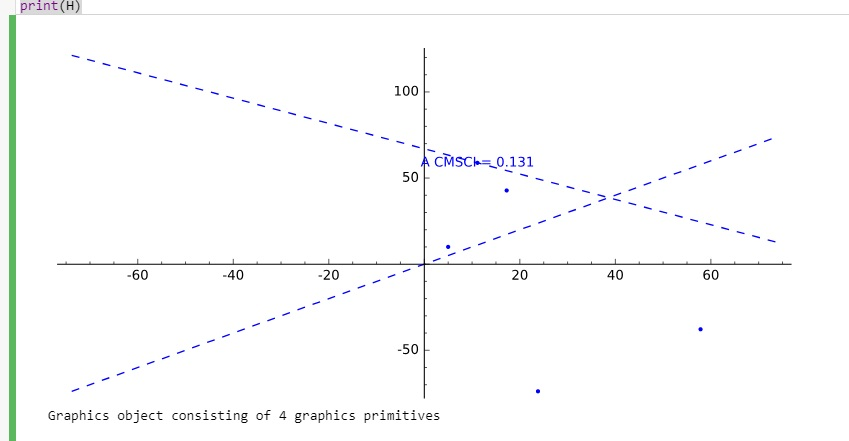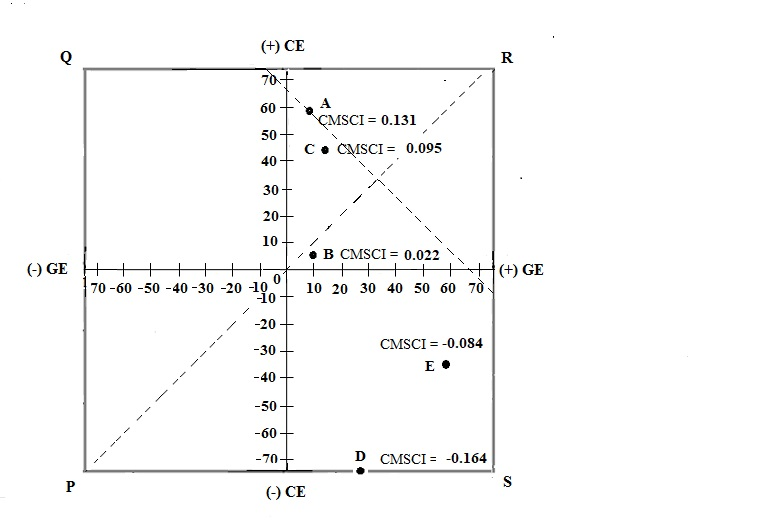# Incorrect graph structure

Hi. I have done some research on how to build my graph based on the info i obtained from http://doc.sagemath.org/html/en/refer...

Here is what I have done :

h=plot(x, (x,-73.83,73.83),linestyle="--")
g=plot(-0.7350*x+67, (x,-73.83,73.83),linestyle="--")
r=list_plot([(11.13,58.82),(4.99,10.03),(17.24,42.79),(23.80,-73.83),(57.84,-37.82)], axes_labels=['(+) GE','(+) CE']))
r += text('A CMSCI = 0.131', (11.135, 58.82))
H = h + g + r;H
print(H)


which gave me this below:It looks almost the same as how i want it to be which is (at least the lines and the points are there (haha)):Few questions here:

1. how to scale the axis properly. my y-axis is wider than x-axis
2. how to form the outer box and add P, Q, R, S labels
3. i manage to form the axis title only for (+)GE and (+)CE, how about the (-) ones?

Thank you.

edit retag close merge delete

Sort by » oldest newest most voted

Getting the same scale for the x and y axes is achieved using aspect_ratio.

Getting an outer box is achieved using frame. The ticks will be on the box though.

Not sure how to get the labels on the min side of the axes...

The following is maybe already a step closer to your desired output.

h = plot(x, (x, -73.83, 73.83), linestyle="--")
g = plot(-0.7350*x + 67, (x, -73.83, 73.83), linestyle="--")
p = [(11.13, 58.82), (4.99, 10.03), (17.24, 42.79), (23.80, -73.83), (57.84, -37.82)]
r = list_plot(p, axes_labels=['(+) GE', '(+) CE'])
r += text('A CMSCI = 0.131', (11.135, 58.82))
H = h + g + r
H.show(aspect_ratio=1, frame=True, ymin= -73.83, ymax=73.83)


See the possible options to show in the documentation, either by typing H.show? after defining H as above, or online at:

more

Thank you for the reply. Just a quick question: How to fix the y-axis range? I manage to fix the range for x-axis by using: , (x,-73.83,73.83), but couldn't do so for y-axis. The reason here is because I need a square box.

I found this in the documentation you gave :

L = line([(1,2), (3,-4), (2, 5), (1,2)])

L.set_axes_range(-1, 20, 0, 2)

Tried it but it did not quite worked. It said:

AttributeError: 'list' object has no attribute 'set_axes_range'

1

I edited the answer, adding ymin=..., ymax=....

thank you for your help. This gave me the graph i want. thanks.